The “forecastHybrid” package provides functions to build composite models using multiple individual component models from the “forecast” package. These `hybridModel` objects can then be manipulated with many of the familiar functions from the “forecast” and “stats” packages including `forecast()`, `plot()`, `accuracy()`, `residuals()`, and `fitted()`.

Installation

The stable release of the package is hosted on CRAN and can be installed as usual.

``````install.packages("forecastHybrid")
``````

The latest development version can be installed using the “devtools” package.

``````devtools::install_github("ellisp/forecastHybrid/pkg")
``````

Version updates to CRAN will be published frequently after new features are implemented, so the development version is not recommended unless you plan to modify the code.

Basic usage

``````library(forecastHybrid)
``````

Quick start

If you don't have time to read the whole guide and want to get started immediately with sane default settings to forecast the `USAccDeaths` timeseries, run the following:

``````quickModel <- hybridModel(USAccDeaths)
``````
``````## Fitting the auto.arima model
``````
``````## Fitting the ets model
``````
``````## Fitting the thetam model
``````
``````## Fitting the nnetar model
``````
``````## Fitting the stlm model
``````
``````## Fitting the tbats model
``````
``````forecast(quickModel)
``````
``````##          Point Forecast    Lo 80     Hi 80    Lo 95     Hi 95
## Jan 1979       8337.989 7888.670  8892.519 7530.555  9172.368
## Feb 1979       7509.460 6748.117  8035.616 6404.944  8322.696
## Mar 1979       8238.747 7178.993  8886.679 6904.007  9146.115
## Apr 1979       8527.886 7553.726  9194.629 7285.827  9500.477
## May 1979       9340.375 8165.932 10112.349 7847.051 10442.376
## Jun 1979       9772.366 8467.777 10525.745 8160.541 10878.297
## Jul 1979      10674.015 9171.578 11613.448 8850.177 11987.171
## Aug 1979      10003.006 9051.371 10830.331 8773.748 11224.086
## Sep 1979       9010.566 8281.314  9944.791 7892.801 10357.609
## Oct 1979       9250.704 8270.437 10198.510 8028.956 10629.548
## Nov 1979       8778.732 8018.969  9732.246 7589.183 10180.765
## Dec 1979       9097.898 8255.912 10255.628 7816.759 10720.971
## Jan 1980       8364.153 7485.300  9496.297 7033.420 10011.749
## Feb 1980       7563.401 6687.306  8749.398 6141.503  9295.201
## Mar 1980       8256.782 6950.696  9586.522 6616.604 10161.075
## Apr 1980       8540.570 7340.046  9940.466 7035.628 10542.396
## May 1980       9334.268 7798.001 10861.976 7480.817 11490.092
## Jun 1980       9762.914 8145.340 11280.306 7805.118 11933.559
## Jul 1980      10636.111 8555.569 12373.743 8146.373 13051.201
## Aug 1980       9961.161 8594.201 11596.927 8239.017 12297.755
## Sep 1980       8996.012 7949.455 10718.099 7261.423 11441.542
## Oct 1980       9238.086 7977.186 10978.824 7417.369 11724.196
## Nov 1980       8782.225 7623.215 10519.779 6856.540 11286.453
## Dec 1980       9089.979 7951.715 11050.531 7288.262 11837.932
``````
``````plot(forecast(quickModel), main = "Forecast from auto.arima, ets, thetam, nnetar, stlm, and tbats model")
``````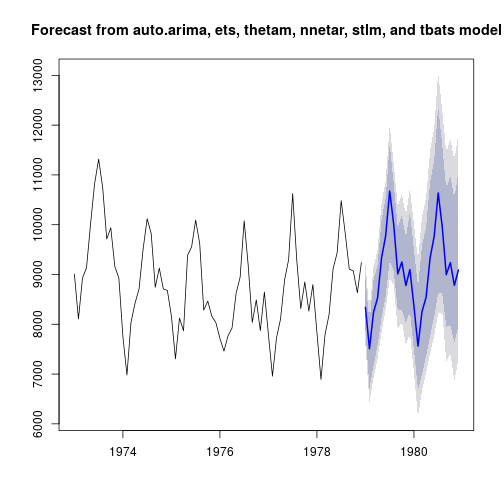Fitting a model

The workhorse function of the package is `hybridModel()`, a function that combines several component models from the “forecast” package. At a minimum, the user must supply a `ts` or `numeric` vector for `y`. In this case, the ensemble will include all six component models: `auto.arima()`, `ets()`, `thetam()`, `nnetar()`, `stlm()`, and `tbats()`. To instead use only a subset of these models, pass a character string to the `models` argument with the first letter of each model to include. For example, to build an ensemble model on a simulated dataset with `auto.arima()`, `ets()`, and `tbats()` components, run

``````# Build a hybrid forecast on a simulated dataset using auto.arima, ets, and tbats models.
# Each model is given equal weight
set.seed(12345)
series <- ts(rnorm(18), f = 2)
hm1 <- hybridModel(y = series, models = "aet", weights = "equal")
``````
``````## Fitting the auto.arima model
``````
``````## Fitting the ets model
``````
``````## Fitting the tbats model
``````

The individual component models are stored inside the `hybridModel` objects and can viewed in their respective slots, and all the regular methods from the “forecast” package could be applied to these individual component models.

``````# View the individual models
hm1\$auto.arima
``````
``````## Series: y
## ARIMA(0,0,0) with zero mean
##
## sigma^2 estimated as 0.6659:  log likelihood=-21.88
## AIC=45.76   AICc=46.01   BIC=46.65
``````
``````# See forecasts from the auto.arima model
plot(forecast(hm1\$auto.arima))
``````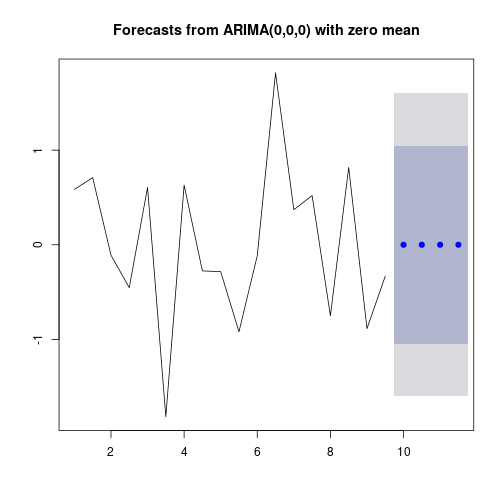Model diagnostics

The `hybridModel()` function produces an S3 object of class `forecastHybrid`.

``````class(hm1)
``````
``````##  "hybridModel"
``````
``````is.hybridModel(hm1)
``````
``````##  TRUE
``````

The `print()` and `summary()` methods print information about the ensemble model including the weights assigned to each individual component model.

``````print(hm1)
``````
``````## Hybrid forecast model comprised of the following models: auto.arima, ets, tbats
## ############
## auto.arima with weight 0.333
## ############
## ets with weight 0.333
## ############
## tbats with weight 0.333
``````
``````summary(hm1)
``````
``````##            Length Class  Mode
## auto.arima 18     ARIMA  list
## ets        19     ets    list
## tbats      21     bats   list
## weights     3     -none- numeric
## frequency   1     -none- numeric
## x          18     ts     numeric
## xreg        1     -none- list
## models      3     -none- character
## fitted     18     ts     numeric
## residuals  18     ts     numeric
``````

Two types of plots can be created for the created ensemble model: either a plot showing the actual and fitted value of each component model on the data or individual plots of the component models as created by their regular S3 `plot()` methods. Note that a `plot()` method does not exist in the “forecast” package for objects generated with `stlm()`, so this component model will be ignored when `type = "models"`, but the other component models will be plotted regardless.

``````plot(quickModel, type = "fit")
``````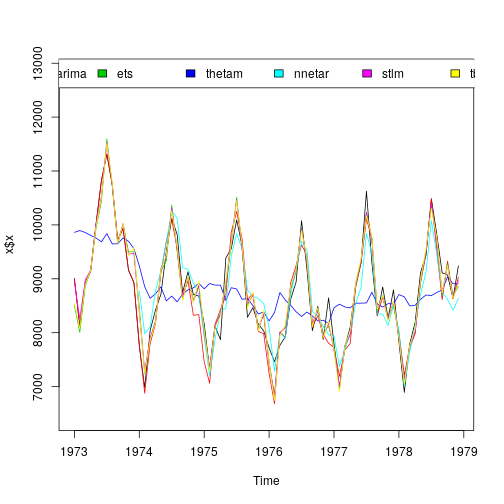``````plot(quickModel, type = "models")
``````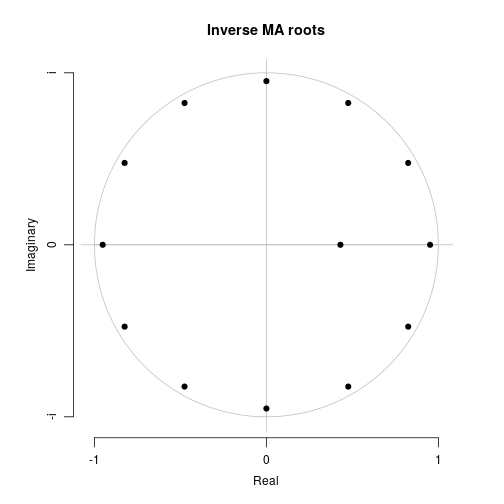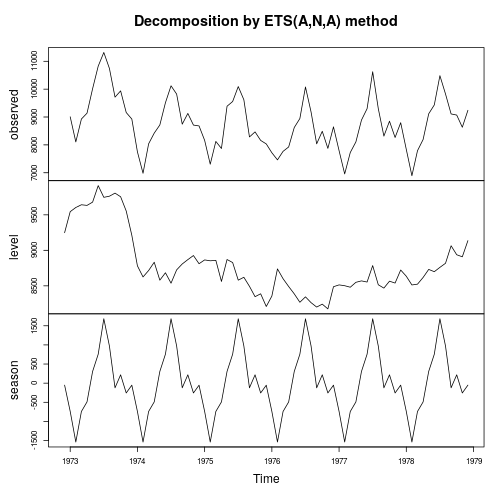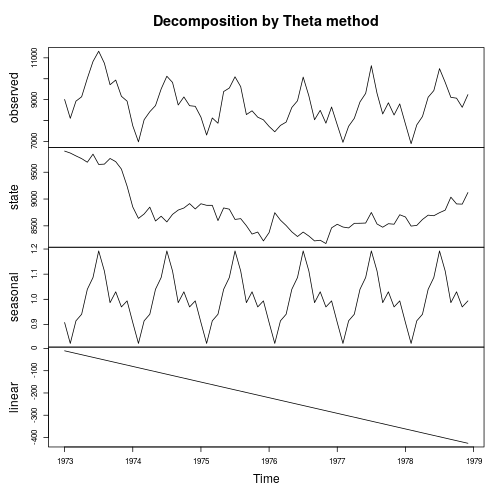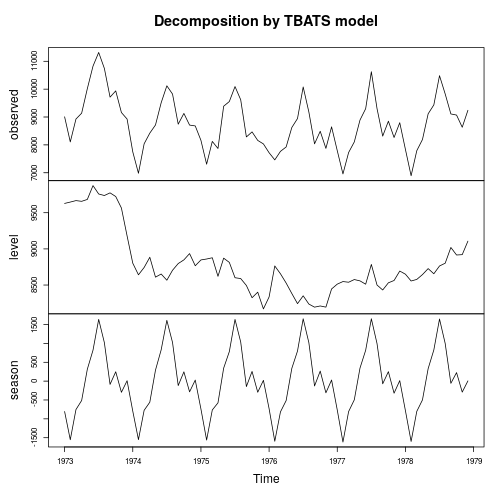Since version 0.4.0, `ggplot` graphs are available. Note, however, that the `nnetar`, and `tbats` models do not have `ggplot::autoplot()` methods, so these are not plotted.

``````plot(quickModel, type = "fit", ggplot = TRUE)
``````
``````## Warning: Removed 12 rows containing missing values (geom_path).
``````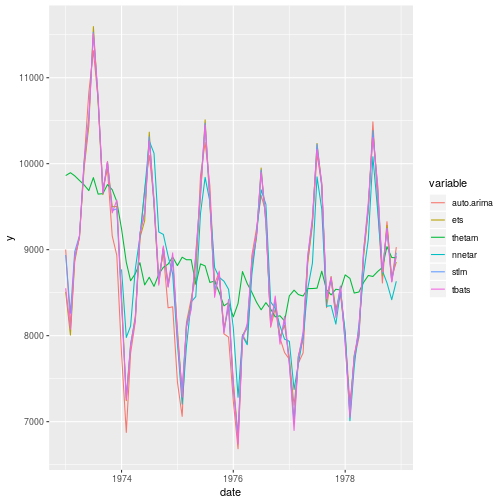``````plot(quickModel, type = "models", ggplot = TRUE)
``````

By default each component model is given equal weight in the final ensemble. Empirically this has been shown to give good performance in ensembles [see @Armstrong2001], but alternative combination methods are available: the inverse root mean square error (`RMSE`), inverse mean absolute error (`MAE`), and inverse mean absolute scaled error (`MASE`). To apply one of these weighting schemes of the component models, pass this value to the `errorMethod` argument and pass either `"insample.errors"` or `"cv.errors"` to the `weights` argument.

``````hm2 <- hybridModel(series, weights = "insample.errors", errorMethod = "MASE", models = "aenst")
``````
``````## Warning in hybridModel(series, weights = "insample.errors", errorMethod =
## "MASE", : Using insample.error weights is not recommended for accuracy and
## may be deprecated in the future.
``````
``````## Fitting the auto.arima model
``````
``````## Fitting the ets model
``````
``````## Fitting the nnetar model
``````
``````## Fitting the stlm model
``````
``````## Fitting the tbats model
``````
``````hm2
``````
``````## Hybrid forecast model comprised of the following models: auto.arima, ets, nnetar, stlm, tbats
## ############
## auto.arima with weight 0.164
## ############
## ets with weight 0.164
## ############
## nnetar with weight 0.306
## ############
## stlm with weight 0.173
## ############
## tbats with weight 0.194
``````

After the model is fit, these weights are stored in the `weights` attribute of the model. The user can view and manipulated these weights after the fit is complete. Note that the `hybridModel()` function automatically scales weights to sum to one, so a user should similar scale the weights to ensure the forecasts remain unbiased. Furthermore, the vector that replaces `weights` must retain names specifying the component model it corresponds to since weights are not assigned by position but rather by component name. Similarly, individual components may also be replaced

``````hm2\$weights
``````
``````## auto.arima        ets     nnetar       stlm      tbats
##  0.1637556  0.1635812  0.3058342  0.1732488  0.1935802
``````
``````newWeights <- c(0.1, 0.2, 0.3, 0.1, 0.3)
names(newWeights) <- c("auto.arima", "ets", "nnetar", "stlm", "tbats")
hm2\$weights <- newWeights
hm2
``````
``````## Hybrid forecast model comprised of the following models: auto.arima, ets, nnetar, stlm, tbats
## ############
## auto.arima with weight 0.1
## ############
## ets with weight 0.2
## ############
## nnetar with weight 0.3
## ############
## stlm with weight 0.1
## ############
## tbats with weight 0.3
``````
``````hm2\$weights <- 0.2
hm2\$weights <- 0.1
hm2
``````
``````## Hybrid forecast model comprised of the following models: auto.arima, ets, nnetar, stlm, tbats
## ############
## auto.arima with weight 0.2
## ############
## ets with weight 0.1
## ############
## nnetar with weight 0.3
## ############
## stlm with weight 0.1
## ############
## tbats with weight 0.3
``````

This `hybridModel` S3 object can be manipulated with the same familiar interface from the “forecast” package, including S3 generic functions such as `accuracy`, `forecast`, `fitted`, and `residuals`.

``````# View the first 10 fitted values and residuals
``````
``````## Time Series:
## Start = c(1, 1)
## End = c(3, 2)
## Frequency = 2
##  0.012621269 0.010804126 0.008561907 0.008984650 0.010541407 0.008636514
``````
``````head(residuals(hm1))
``````
``````## Time Series:
## Start = c(1, 1)
## End = c(3, 2)
## Frequency = 2
##  0.012621269 0.010804126 0.008561907 0.008984650 0.010541407 0.008636514
``````

In-sample errors and various accuracy measure can be extracted with the `accuracy` method. The “forecastHybrid” package creates an S3 generic from the `accuracy` method in the “forecast” package, so `accuracy` will continue to function as normal with objects from the “forecast” package, but now special functionality is created for `hybridModel` objects. To view the in-sample accuracy for the entire ensemble, a simple call can be made.

``````accuracy(hm1)
``````
``````##                    ME      RMSE       MAE      MPE     MAPE       ACF1
## Test set -0.006167957 0.8146777 0.6675259 101.5092 101.5092 -0.2202369
##          Theil's U
## Test set 0.9281098
``````

In addition to retrieving the ensemble's accuracy, the individual component models' accuracies can be easily viewed by using the `individual = TRUE` argument.

``````accuracy(hm1, individual = TRUE)
``````
``````## \$auto.arima
##                       ME      RMSE       MAE MPE MAPE      MASE       ACF1
## Training set 0.006161102 0.8159991 0.6667319 100  100 0.7381739 -0.2202409
##
## \$ets
##                         ME      RMSE       MAE      MPE     MAPE      MASE
## Training set -6.878921e-05 0.8160167 0.6674428 100.7412 100.7412 0.7389609
##                    ACF1
## Training set -0.2202415
##
## \$tbats
##                       ME     RMSE       MAE      MPE     MAPE      MASE
## Training set -0.02459618 0.812366 0.6684029 103.7863 103.7863 0.6244447
##                    ACF1
## Training set -0.2201724
``````

Forecasting

Now's let's forecast future values. The `forecast()` function produce an S3 class `forecast` object for the next 48 periods from the ensemble model.

``````hForecast <- forecast(hm1, h = 48)
``````

Now plot the forecast for the next 48 periods. The prediction intervals are preserved from the individual component models and currently use the most extreme value from an individual model, producing a conservative estimate for the ensemble's performance.

``````plot(hForecast)
``````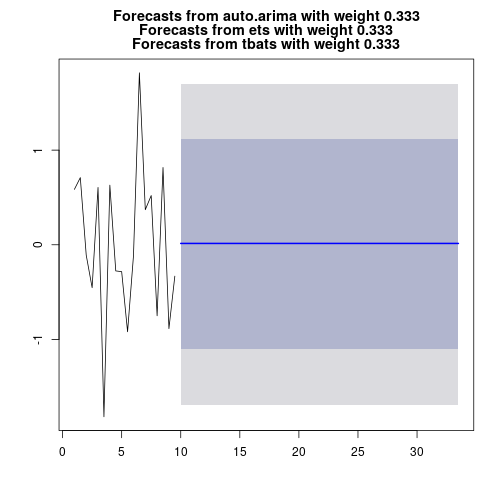The package aims to make fitting ensembles easy and quick, but it still allows advanced tuning of all the parameters available in the “forecast” package. This is possible through usage of the `a.args`, `e.args`, `n.args`, `s.args`, and `t.args` lists. These optional list arguments may be applied to one, none, all, or any combination of the included individual component models. Consult the documentation in the “forecast” package for acceptable arguments to pass in the `auto.arima`, `ets`, `nnetar`, `stlm`, and `tbats` functions.

``````hm3 <- hybridModel(y = series, models = "aefnst",
a.args = list(max.p = 12, max.q = 12, approximation = FALSE),
n.args = list(repeats = 50),
s.args = list(robust = TRUE),
t.args = list(use.arma.errors = FALSE))
``````
``````## Fitting the auto.arima model
``````
``````## Fitting the ets model
``````
``````## Fitting the thetam model
``````
``````## Fitting the nnetar model
``````
``````## Fitting the stlm model
``````
``````## Fitting the tbats model
``````

Since the `lambda` argument is shared between most of the models in the “forecast” framework, it is included as a special parameter that can be used to set the Box-Cox transform in all models instead of settings this individually. For example,

``````hm4 <- hybridModel(y = wineind, models = "ae", lambda = 0.15)
``````
``````## Fitting the auto.arima model
``````
``````## Fitting the ets model
``````
``````hm4\$auto.arima\$lambda
``````
``````##  0.15
##  FALSE
``````
``````hm4\$ets\$lambda
``````
``````##  0.15
##  FALSE
``````

Users can still apply the `lambda` argument through the tuning lists, but in this case the list-supplied argument overwrites the default used across all models. Compare the following two results.

``````hm5 <- hybridModel(y = USAccDeaths, models = "aens", lambda = 0.2,
a.args = list(lambda = 0.5),
n.args = list(lambda = 0.6))
``````
``````## Fitting the auto.arima model
``````
``````## Fitting the ets model
``````
``````## Fitting the nnetar model
``````
``````## Fitting the stlm model
``````
``````hm5\$auto.arima\$lambda
``````
``````##  0.5
##  FALSE
``````
``````hm5\$ets\$lambda
``````
``````##  0.2
##  FALSE
``````
``````hm5\$nnetar\$lambda
``````
``````##  0.6
``````
``````hm5\$stlm\$lambda
``````
``````##  0.2
##  FALSE
``````

Note that lambda has no impact on `thetam` models, and that there is no `f.args` argument to provide parameters to `thetam`. Following `forecast::thetaf` on which `thetam` is based, there are no such arguments; it always runs with the defaults.

Covariates can also be supplied to `auto.arima` and `nnetar` models as is done in the “forecast” package. To do this, utilize the `a.args` and `n.args` lists. Note that the `xreg` may also be passed to a `stlm` model, but only when `method = "arima"` instead of the default `method = "ets"`. Unlike the usage in the “forecast” package, the `xreg` argument should be passed as a matrix, not a dataframe. The `stlm` models require that the input series will be seasonal, so in the example below we will convert the input data to a `ts` object. If a `xreg` is used in training, it must also be supplied to the `forecast()` function in the `xreg` argument. Note that if the number of rows in the `xreg` to be used for the forecast does not match the supplied `h` forecast horizon, the function will overwrite `h` with the number of rows in `xreg` and issue a warning.

``````# Use the beaver1 dataset with the variable "activ" as a covariate and "temp" as the time series
# Divice this into a train and test set
trainSet <- beaver1[1:100, ]
testSet <- beaver1[-(1:100), ]
trainXreg <- matrix(trainSet\$activ)
testXreg <- matrix(testSet\$activ)

# Create the model
beaverhm <- hybridModel(ts(trainSet\$temp, f = 6),
models = "aenst",
a.args = list(xreg = trainXreg),
n.args = list(xreg = trainXreg),
s.args = list(xreg = trainXreg, method = "arima"))
``````
``````## Fitting the auto.arima model
``````
``````## Fitting the ets model
``````
``````## Fitting the nnetar model
``````
``````## Fitting the stlm model
``````
``````## Fitting the tbats model
``````
``````# Forecast future values
beaverfc <- forecast(beaverhm, xreg = testXreg)

# View the accuracy of the model
accuracy(beaverfc, testSet\$temp)
``````
``````##                        ME       RMSE        MAE         MPE      MAPE
## Training set 0.0008460645 0.08323059 0.05587866 0.001903305 0.1513580
## Test set     0.0701079432 0.10446638 0.08149130 0.189589078 0.2205923
##                  MASE        ACF1
## Training set 0.847165 -0.01249166
## Test set     1.235473          NA
``````

Cross Validation

It can be useful to perform cross validation on a forecasting model to estimate a model's out-of-sample forecasting performance. The `cvts()` function allows us to do this on arbitrary functions. We could do this as part of a model selection procedure to determine which models to include in our call to `hybridModel()` or merely to understand how well we expect to forecast the series during unobserved windows.

For example, let's perform cross validation for a `stlm()` model and a `naive()` model on the `woolyrnq` time series. The most important `cvts()` arguments that commonly need adjusting are `rolling` (if `TRUE`, the model will always be fit on a fixed `windowSize` instead of growing by one new observation for each new model fit during cross validation), `windowSize` (starting length of time series to fit a model), and `maxHorizon` (the forecast horizon for predictions from each model). Since a naive forecast is a good baseline that any decent model should surpass, let's see how the `stlm()` model compares.

``````stlmMod <- cvts(woolyrnq, FUN = stlm, windowSize = 100, maxHorizon = 8)
naiveMod <- cvts(woolyrnq, FUN = naive, windowSize = 100, maxHorizon = 8)
accuracy(stlmMod)
``````
``````##                             ME      RMSE       MAE
## Forecast Horizon  1  143.26726 276.46300 236.44509
## Forecast Horizon  2 -185.31041 305.09020 242.36353
## Forecast Horizon  3 -269.48582 284.78038 269.48582
## Forecast Horizon  4  -82.66850 121.21761  88.65454
## Forecast Horizon  5 -128.73274 128.89398 128.73274
## Forecast Horizon  6  -66.81041 250.92150 241.86353
## Forecast Horizon  7  -44.48582  58.45955  44.48582
## Forecast Horizon  8  -62.66850 143.10601 128.65454
``````
``````accuracy(naiveMod)
``````
``````##                          ME      RMSE    MAE
## Forecast Horizon  1  -768.5  802.0365  768.5
## Forecast Horizon  2  -229.0  324.5628  230.0
## Forecast Horizon  3   142.5  184.0611  142.5
## Forecast Horizon  4   -88.0  124.4508   88.0
## Forecast Horizon  5 -1040.5 1040.5001 1040.5
## Forecast Horizon  6  -110.5  254.7165  229.5
## Forecast Horizon  7   367.5  367.7479  367.5
## Forecast Horizon  8   -68.0  144.9414  128.0
``````

We see from looking at the accuracy measure–in particular the smaller RMSE and MAE–the `stlm()` model unsurprisingly performs better and will likely give us better future forecasts. We also notice that the apparent edge over the naive forecast tends to diminish or even disappear for longer forecast horizons, and a look at the original time series makes this result obvious: this time series lacks an obvious trend and is a relatively difficult time series to forecast past a fewer seasonal periods, so the naive model will not perform relatively poorly.

``````plot(woolyrnq)
``````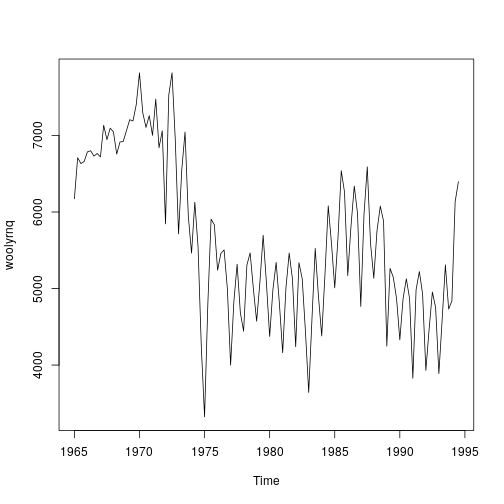We can also use custom functions, for example `fcast()` from the “GMDH” package. We must be very careful that our custom forecast function still produces an expected “forecast” S3 class object and that the ts object start, end, and frequency properties are preserved.

``````library(GMDH)
GMDHForecast <- function(x, h){
fc <- GMDH::fcast(x, f.number = h)
# GMDH doesn't produce a ts object with correct attributes, so we build it
end <- tsp(x)
freq <- frequency(x)
# Set the correct start, end, and frequency for the ts forecast object
tsProperties <- c(end + 1 / freq, end + h / freq, freq)
tsp(fc\$mean) <- tsProperties
tsp(fc\$upper) <- tsProperties
tsp(fc\$lower) <- tsProperties
class(fc) <- "forecast"
return(fc)
}
series <- subset(woolyrnq, end = 12)
gmdhcv <- cvts(series, FCFUN = GMDHForecast, windowSize = 10, maxHorizon = 1)
``````

As a final example, suppose we foolish want to implement our own version of `naive()` for performing cross validation. The `FUN` and `FCFUN` could then look like

``````customMod <- function(x){
result <- list()
result\$series <- x
result\$last <- tail(x, n = 1)
class(result) <- "customMod"
return(result)
}
forecast.customMod <- function(x, h = 12){
result <- list()
result\$model <- x
result\$mean <- rep(x\$last, h)
class(result) <- "forecast"
return(result)
}
series <- subset(AirPassengers, end = 94)
``````

cvobj <- cvts(series, FUN = customMod, FCFUN = forecast.customMod)

Cross Validation Weights

Previously we explored fitting `hybridModel()` objects with `weights = "equal"` or `weights = "insample.errors`, but we can now leverage the process conducted in `cvts()` to select the appropriate weights intelligently based on the expected out-of-sample forecast accuracy of each component model. While this is the methodologically-sound weight procedure, it also comes at significant computational cost since the cross validation procedure necessitates fitting each model several times for each cross validation fold in addition to the final fit on the whole dataset. Fortunately this process can be conducted in parallel if multiple cores are available. Some of the arguments explained above in `cvts()` such as `windowSize` and the `cvHorizon` can also be controlled here.

``````cvMod <- hybridModel(woolyrnq, models = "ns", weights = "cv.errors", windowSize = 100, cvHorizon = 8, num.cores = 4)
cvMod
``````